LAB #1

Introduction to Spectrophotometry

"Properties of Light"...pg.168-170. Stop @ "Light absorption and biological..."
Bring a calculator to lab

Introduction

The purposes of this laboratory are to introduce you to:

1. Conventions used in making solutions; molarity, and per cent.
2. Spectrophotometry and the use of the microplate reader.
3. Procedures for obtaining, recording, and analyzing data.
4. Conventions used in presenting data in graphs.
5. Procedures for planning and working through a series of related experiments.

Note: Remember to put all of your data, answers to questions and problems, and notes on the blank pages of this lab manual. Keep it beside you and write in it as you work.

Concentrations of solutions
It is important that you understand the units of the metric system (i.e. milli- and micro-). If you do not, please review these units of measurement as well as the Celsius (centigrade) temperature scale in the back of your textbook (Appendix 3).
In the instructions below, the solute is the substance dissolved, the solvent is the liquid in which the solute is dissolved, and the resulting mixture is the solution.

Molar Concentrations
In technical terms, a mole of a compound is 6.02 x 10^23 molecules of that compound. Practically speaking, a mole is its molecular weight in grams. A one molar (1.0 M) solution has one mole of a solute dissolved in 1000 ml (one liter) of solvent. The molecular weight of NaCl is 58.54. Therefore:

1.0 M solution of NaCl has 58.54 g NaCl dissolved in dH
20 with a final volume of 1000 ml.
0.1 M solution of NaCl has 5.85 g in 1 liter - or 0.585 g in 100 ml - or 0.058 g in 10 ml.
0.2 M solution of NaCl has 11.71 g in 1 liter - or 1.17 g in 100 ml - or 0.117 g in 10 ml.

Concentration in Percent (Weight/Volume = w/v)
By definition, percent means "in a hundred" and by convention, a 10% w/v solution contains 10 grams of a solute in a total volume of 100 ml of the solution. A w/v solution is not made by adding 10 grams of a solute to 100 ml of solvent, but instead by dissolving 10 grams solute in enough solvent to reach a total volume of 100 ml of the solution. Note the differences in results as you think through the following mental exercise.

Experiment 1:

Here is a demonstration experiment for you to observe by reading the directions and examining the graduated cylinder at the front bench. The directions below were followed and you should record the results in your lab manual.

Step number one has been done for you:
1. Measure 5 grams (g) of sodium chloride (NaCl) was obtained and added to exactly 50 ml of distilled water (dH20) in a graduated cylinder. The salt was stirred vigorously to dissolve it.
2. Determine the excess volume by reading the volume of the graduated cylinder. This was a failed attempt to make a 10% w/v solution. The number of ml in excess of 50 represents the volume displaced by the 5 g of dissolved NaCl.
3. What is the actual percentage of NaCl (w/v)?

Concentrations in Percent (Volume/Volume = v/v)
Aqueous solutes may be specified as percent solutions volume/volume (v/v). One hundred ml of a 5% v/v solution will have 5 ml solute diluted to 100 ml with solvent. Thus a 5% v/v aqueous solution of ethanol is made by diluting 5 ml 100% ethanol with enough dH
20 (95 ml) to make a total of 100 ml (or by diluting 5 liters 100% ethanol with 95 liters dH20).

Experiment 2:

On the front bench is a stock solution (1.0 M) of a dye, neutral red. Make up 2 ml of a 4% v/v solution from this neutral red stock using distilled water (dH
20). Label your tube and save this solution; you will use it later today.

III. Spectrophotometry.

A solution, such as neutral red, appears colored because it absorbs certain wavelengths of light in the visible spectrum and transmits or reflects others. Each solution with a different solute has its own characteristic properties or "spectrum." A spectrophotometer is an optical machine that measures and lets you see (sense) how much light energy is transmitted by a substance in solution at different wavelengths of radiant energy. Biologists use the spectrophotometer for two different purposes (we will do both in the laboratory today):

1. to determine the absorption spectrum of a pure substance in solution, and
2. to determine the concentration of a solution.

A spectrophotometer consists of (in order) a white light source (light of all visible wavelengths), a prism or diffraction grating that separates the light into different wavelengths, a slit through which a narrow beam of the desired wavelength passes (the incident light, I0), a sample solution holder, a photosensitive tube which measures the energy of light transmitted through the solution (I), and a recording device that displays the amount of transmitted light energy digitally or on a dial. See Fig. 1 below.Figure 1. A schematic diagram of the components of a spectrophotometer 20. The arrows indicate the pathway of light.

Transmittance is the ratio of the transmitted light energy (I) to the incident light energy (Io); percent transmittance is 100X that ratio. Transmittance, however, is not proportional to solute concentration, so it is usually converted into absorbance which is proportional to solute concentration. Digital spectrophotometers have readouts for both percent trasmittance and absorbance, but we will always measure the absorbance.

%T = (I ÷ Io)*100

Abs. = log 10 (100/%T)

Above is a simplified driagram of a spectrophotometer that can measure one sample at a time. In our labs, we will be using a microplate reader that is capable of measuring the absorbance of 96 samples in about 8 seconds. The basic design is the same; a selected wavelength of light passes through the samples and a phototube measures the amount of light transmitted through the sample, which the plate reader converts to absorbance. However, the samples are located in microwells that are arranged in an 8 x 12 matrix in one plastic plate (see figure 2). You can put your samples in any or all of the microwells. The plate is moved over an array of 8 fiber optics light sources and 8 phototubes. Each row of 8 is scanned and then the plate advances by one row and the process continues until all 12 rows are scanned. The absorbance data are then displayed on a screen in an 8 x 12 array. These data can be saved in the memory to be printed later. This technology is based on the same principles as older spectrophotometers, but now we can measure more samples in less time. You can also program the plate reader to measure the absorbance of all 96 samples at time intervals of your choice (e.g. every 30 seconds). You should take advantage of these capabilities when you design your experiments.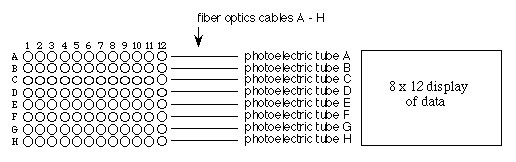Figure 2. Schematic diagram of a microplate reader. Samples are placed in the 96 microwells, analyzed by the 8 channel spectrophotometer, and the absorbance data are displayed in the large LED window.

The Absorption Spectrum

Since solutions of pure substances do not absorb the energy of all wavelengths of light equally, a substance may be identified by the unique pattern of wavelengths absorbed. The chlorophylls in plants absorb strongly in the blue wavelengths (about 450 nm) and red wavelengths (about 650 nm) but reflect the green wavelengths (about 525 nm). A plot of absorbance versus visible wave lengths (400 to 700 nm) for a solution of chlorophyll a shows two major peaks, one at 450 and one at 650 nm, and a valley from 500 to 625 nm (See Figure 3. below) This spectrum is characteristic for chlorophyll a and may be used as an aid in its identification.
By measuring the absorbance of an uncharacterized solution over a range of wavelengths and plotting the absorbance value on the Y-axis and the wavelength on the X-axis, one can determine the absorption spectrum of a sample. The absorption maximum of any pure substance in solution is the wavelength where absorption is the greatest.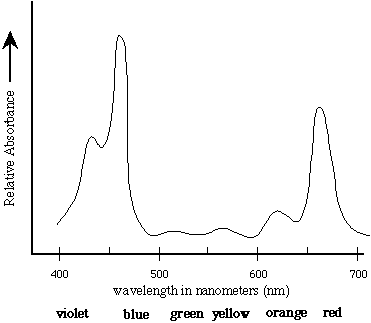Figure 3. The absorption spectrum of chlorophyll a. The absorbance of visible light by chlorophyll a is measured spectrophotometrically as a function of wave lengh. The absorption maximum is about 460 nm.

IV How to use a spectrophotometer for Answer Biological Questions

Standard Concentration Curve and Determining the Concentration of a Characterized Substance

One constructs a standard concentration curve of a solution by measuring the absorbance of several different known concentrations of the solution and graphing the results by plotting absorbance on the Y-axis and concentration on the X-axis.

Spectrophotometry can be used to measure the absolute or relative concentration of a characterized substance in solution. To determine the absolute concentration of a pure substance, one first constructs a standard concentration curve from known concentrations and then takes the absorbance reading of the unknown concentration. The unknown concentration can be determined from the standard curve by drawing a horizontal line on the graph parallel to the X-axis and through the point on the Y-axis which corresponds to the absorbance. This line will intersect the standard curve; at this intersection, a vertical line is dropped to the X-axis and the concentration read from the X-axis.

Two factors are important in determining unknown or relative concentrations. The absorption maximum should be used, and absorbance rather than percent transmittance should be plotted because absorbance is directly proportional to concentration and transmittance is not.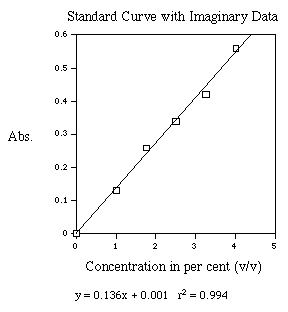Figure 4. This is an example of a standard curve. A best fit line has been generated and the resulting equation and r-squared value are shown below the X-axis label.

Experiment 3:

Using the 4% (v/v) neutral red solution you prepared in experiment #2, set up the following solutions in microfuge tubes using distilled water available on the lab benches.

TABLE 1. Volumes of neutral red and distilled water used to prepare solutions for tubes 1 - 6. You must calculate the volumes and fill in the table for the first two columns before you begin the experiment.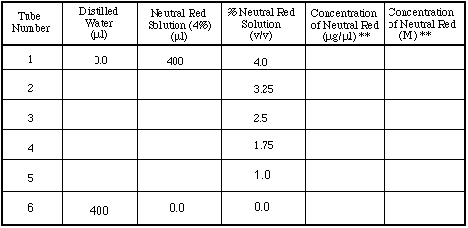** These parts of the table should be completed when you are answering question #6 at the end of the lab.

Experiment 4:

Determination of absorption maximum.
Use tubes 1 - 6.
Put 200 µl of the six different dilutions of neutral red into six different wells (wells H7 - H12) of your 96-well plate.
You will collect the data for experiments 4 and 5 at the same time (see below).

Experiment 5:

Determination of a standard concentration curve for neutral red.

You will generate these data at the same time as those from Experiment 4. Use only the data from the wavelength that is the absorption maximum for neutral red. With the appropriate data, you will generate a curve to measure the unknown concentration of a solution. Follow the directions below to collect the data.

Operation of the Microplate Reader

1. Turn on the spectrophotometer and let it warm up for 15 minutes.

There are two main variables which you must pay attention to while you use the plate reader: the ANALYSIS NUMBER and the FORMAT NUMBER. Format is easy since we will always use format #1, which simply means that all of the wells are to be analyzed for absorbance. Analysis is the list of options you want to use when performing your spectrophometric analysis of your samples. Specifically, you tell the machine which wavelength of light to use, to calculate the absorbance, to use a single wavelength of light, and to make only one reading for each time you press the START key. Today, we will use ANALYSIS NUMBERS 1- 6.

2. Before you collect any data, clear the memory of the plate reader. To do this:
press FUNCTION, CLEAR ALL; when it asks you if this is OK?,
press FUNCTION, CLEAR.

3. Start the reading of your samples by using all six wavelengths of light that the plate reader can use. At the end of each reading, you will get a printout of your absorbance data. When you have finished all six, tear off your paper, clean up any mess you created, remove your 96-well plate, and return to your seats. To execute the analysis with all 6 wavelengths (1 = 340; 2 = 405; 3 = 450; 4 = 490; 5 = 595; and 6 = 655 nm):

a. press ANALYSIS, 1, ENTER, START. When the reading is complete,
b. press ANALYSIS, 2, ENTER, START. When the reading is complete,
c. press ANALYSIS, 3, ENTER, START. When the reading is complete,
d. press ANALYSIS, 4, ENTER, START. When the reading is complete,
e. press ANALYSIS, 5, ENTER, START. When the reading is complete,
f. press ANALYSIS, 6, ENTER, START. When the reading is complete,

After you have completed Experiments 4 and 5:

Analysis of the Data for experiments 4 and 5

Experiment 4:

Now that you have several pages of data, what do you do next? Follow the order of the experiments, begining with number 4; determine the absorption maximum for neutral red. Find the wavelength that produced the highest absorbance values. This is the absorption maximum for neutral red and all subsequent experiments should use only the data generated with this wavelength of light. To generate an abbreviated absorption spectrum, plot the absorbance for tube number 3 in a graph with Y-axis as the absorbance value and the X-axis as the wavelength of light. This will be graph number 1.

Experiment 5:

Using the absorbance data that were generated with the absorption maximum, subtract the absorbance of the blank from the values for the samples. This corrects for the amount of light absorbed by the plastic and the water. Now, construct a graph with the X-axis as per cent neutral red (increasing from left to right), and the Y-axis as absorbance at your selected absorbance maximum (e.g. Abs 666nm) using your adjusted absorbance values. Plot the results from tubes 1 through 6 and draw a straight line (best fit) to generate the standard concentration curve. This will be graph number 2.

After completing these analyses and generating 2 graphs, continue with experiment number 6 below.

Perform Experiment 6:

Determination of the concentration of the unknown neutral red solutions.

Obtain four neutral red solutions of unknown concentration from your instructor and record the identifying letter in your lab manual. Determine the concentration of your unknowns by putting 200 µl of it in a well, and record the well's identifying letter and number (e.g. H3 - H6). Use the plate reader at the wave length of light that is absorbed the best by neutral red. Subtract the absorbance of water from these values and use these to determine their concentrations.

Analysis of the Data for experiment 6

Experiment 6:

Using the standard curve (graph number 2), determine the concentration of your unknown dilutions of neutral red. For each unknown, the unknown concentration can be determined from the standard curve by drawing a horizontal line on the graph parallel to the X-axis and through the point on the Y-axis which corresponds to the absorbance for the unknown. This line will intersect the standard curve; at this intersection, a vertical line is dropped to the X-axis and the concentration read from the X-axis.

After you have completed your analysis of experiment 6, begin with experiment 7 below.

Experiment 7:

Determine the absorption maximum for NADPH.

Over the next two weeks, we will use NADPH and we need to know which wavelength of light to use. You will want to perform a series of experiments similar to what you did in experiments 4 and 5. Record which wavelength is absorbed the best and verify your results with the instructor.

Experiment 8!:

Clean up. Leave your work station as you found it. Although this may seem like a stupid thing to list in a protocol, it is quite important. It is basic lab courtesy to leave the lab as clean as you found it with equipment back in its proper place(s).

Before you leave lab:

Make sure you have recorded all data and observations and plotted both of your graphs for this week:

1) Maximum absorption wave length of Neutral Red,
2) Standard Curve, and determination of concentration of unknown solutions.
3) Determine the absorption maximum for NADPH.

Questions to answer on your own time, but may prove helpful at test time: Answer them in your lab manual.

1. How do you account for the excess volume you observed in Experiment 1?
2. The solution made in experiment 1 does not constitute a 10% w/v solution of sodium chloride but something less than that. Calculate the actual percentage from data.
3. Why do you have to dissolve the solute in a volume of solvent less than the final volume you eventually want? Does it matter if the salt is added first or second to the graduated cylinder?
4. Describe precisely how you made up the 4% v/v solution of neutral red.
5. Does it matter whether the proper number of ml of solute is added first or second (relative to the water) to the graduated cylinder?
6. The aqueous stock solution of the neutral red is a 1.0 M solution. If neutral red has a molecular weight of 100. How many µg/µl are contained in your 4% (v/v) solution? Calculate the concentration of neutral red in each tube (µg/µl) and add this to the table from experiment 3.
7. Describe how to prepare 50 ml of 70% ethyl alcohol when your only source is a stock container of 95% ethyl alcohol.
8. What is the molarity of your 10% w/v NaCl solution?
9. What is the percent concentration of a 2 M NaCl solution?
10. The molecular weight of Na
2C03 is 106. Describe how you would make up 100 ml of a 0.15 M solution.
11. What is the absorbtion maximum for NADPH?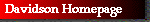© Copyright 2000 Department of Biology, Davidson College, Davidson, NC 28036
Send comments, questions, and suggestions to: macampbell@davidson.edu